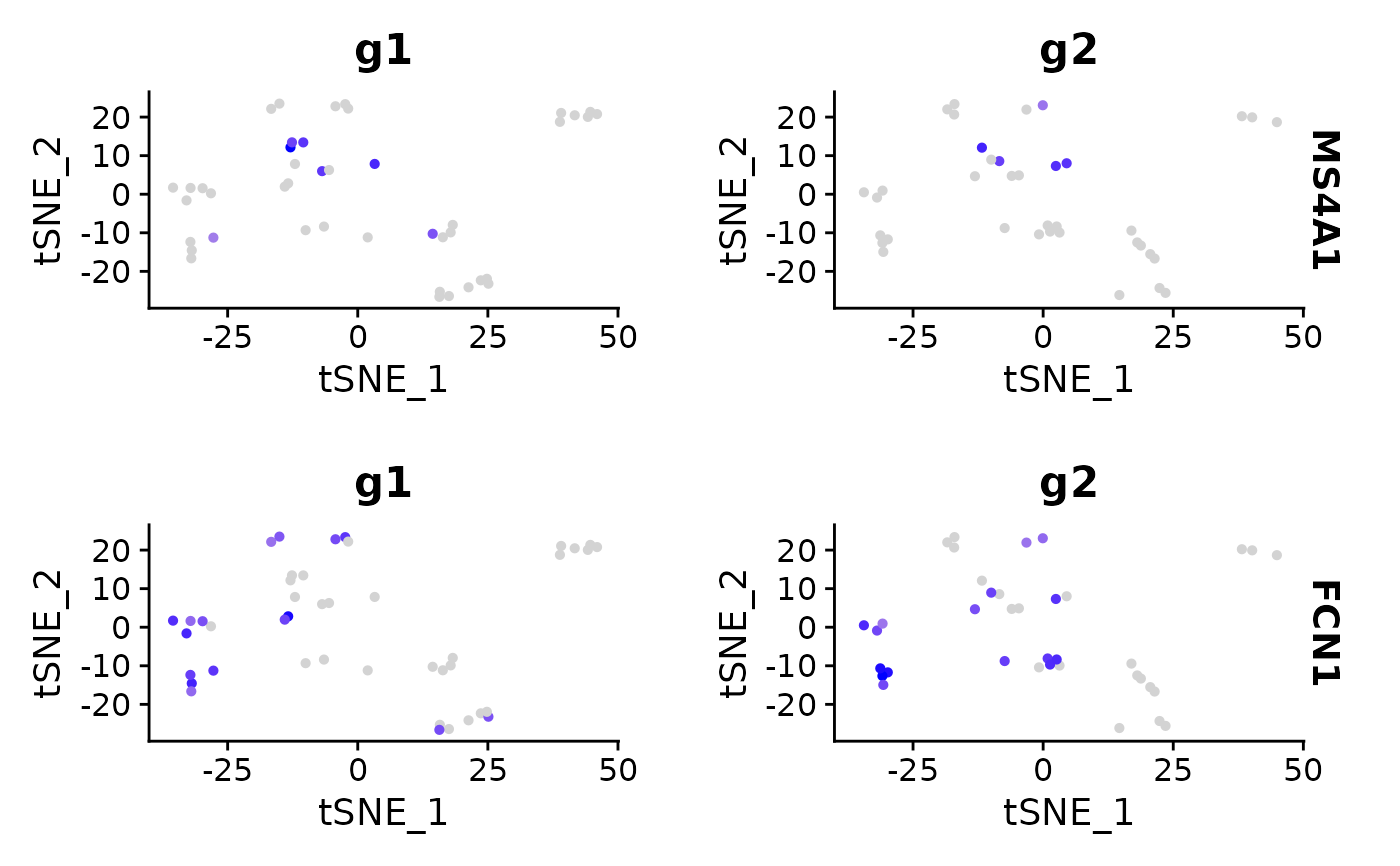Combine ggplot2-based plots into a single plot

CombinePlots(plots, ncol = NULL, legend = NULL, ...)

## Arguments

plots A list of gg objects Number of columns Combine legends into a single legend choose from 'right' or 'bottom'; pass 'none' to remove legends, or NULL to leave legends as they are Extra parameters passed to plot_grid

A combined plot

## Examples

data("pbmc_small")
pbmc_small[['group']] <- sample(
x = c('g1', 'g2'),
size = ncol(x = pbmc_small),
replace = TRUE
)
plot1 <- FeaturePlot(
object = pbmc_small,
features = 'MS4A1',
split.by = 'group'
)
plot2 <- FeaturePlot(
object = pbmc_small,
features = 'FCN1',
split.by = 'group'
)
CombinePlots(
plots = list(plot1, plot2),
legend = 'none',
nrow = length(x = unique(x = pbmc_small[['group', drop = TRUE]]))
)
#> Warning: CombinePlots is being deprecated. Plots should now be combined using the patchwork system.